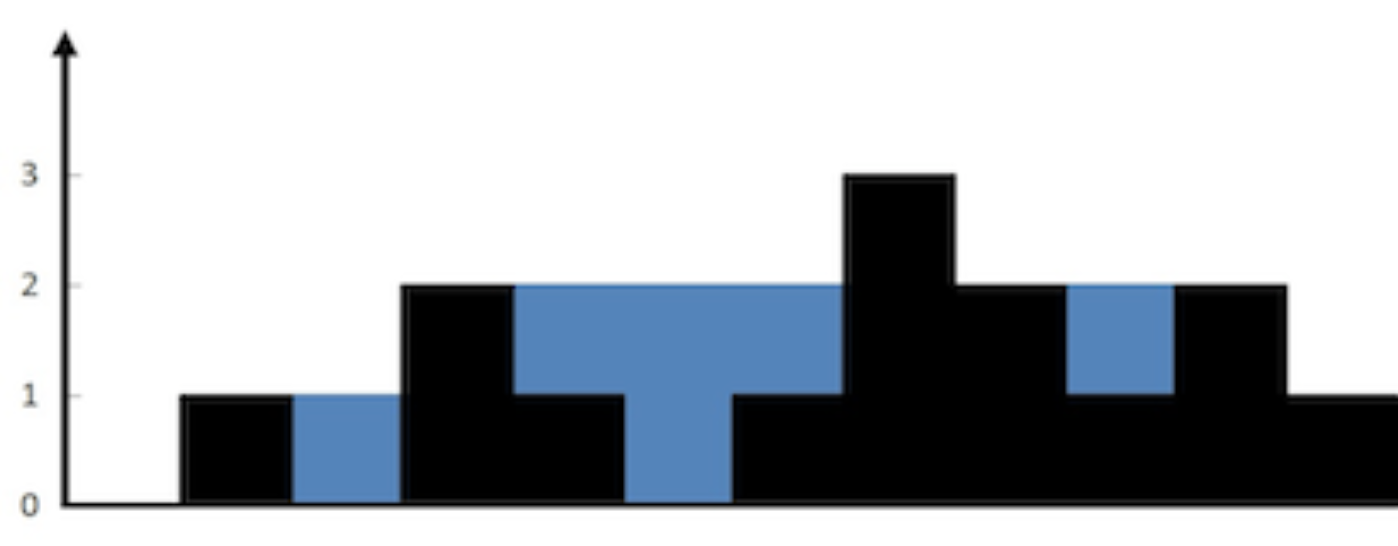# 10 Algorithm Puzzles about Sequences

Some of my favorite problems on LeetCode.Image Source: LeetCode.com

## LC 373 Find K Pairs with Smallest Sums

Given two sorted integer arrays A and B, find the k pairs of (A[i], B[j]) with the smallest sums, where 0≤i<|A|, 0≤j<|B|.

## LC 719 Find K-th Smallest Pair Distance

Given an integer array A of size n, find the k-th smallest distance among all the pairs, where the distance of a pair (A[i], A[j]) is defined as |A[i]-A[j]|.

## LC 239 Sliding Window Maximum

Given an integer array A of size n and a window size k, for each 0≤i<n-k, find the maximum value among A[i], A[i+1], …, A[i+k-1].

## LC 84 Largest Rectangle in Histogram

Given an array A of n non-negative integers, return the maximum value of (j-i+1) * h(i, j) for any 0≤i≤j<n, where h(i, j) is the minimum value among A[i], A[i+1], …, A[j].

## LC 962 Maximum Width Ramp

Given an integer array A of size n, find the largest j-i such that 0≤i<j<n and A[i]≤A[j].

## LC 315 Count of Smaller Numbers After Self

Given an integer array A of size n, count the total number of pairs of A[i] and A[j] such that i<j and A[i]>A[j].

## LC 1674 Minimum Moves to Make Array Complementary

Given a positive integer M and an array A containing n integers in the range [1, M], find the minimum number of elements that need to be changed into another value in [1, M] so that A[i]+A[n-i-1] are equal for all 0≤i<n.

## LC 730 Count Different Palindromic Subsequences

Given a string S that consists of n letters among {‘a’, ‘b’, ‘c’, ‘d’}, count the number of distinct palindromic subsequences of it.

## LC 1147 Longest Chunked Palindrome Decomposition

Given a string S of length n, return the maximum number m such that S can be split into an array A of m substrings and for each 0≤i<m, A[i]=A[m-1-i].

## LC 1397 Find All Good Strings

Given strings S₁ and S₂ of the same length n, and an evil string P of length m, count the number of good strings that are in the lexicographical range [S₁, S₂]. A string is good if it doesn’t contain P as a substring.

Software Engineer, Bookworm

## More from Devin Zuo

Software Engineer, Bookworm